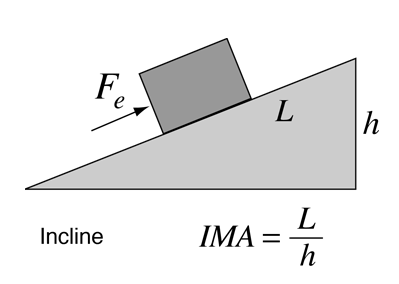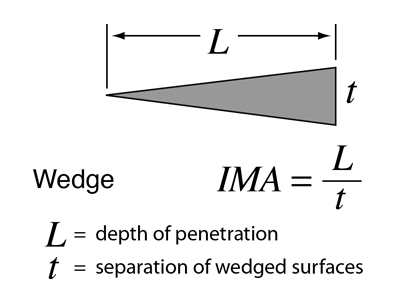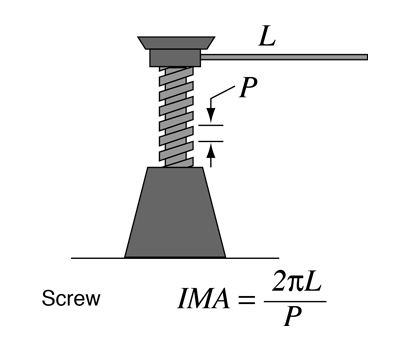# The InclineThe incline is one of the so-called "simple machines" from which many more complex machines are derived. By pushing an object up a slanted surface, one can move the object to height h with a smaller force than the weight of the object. If there were no friction, then the mechanical advantage could be determined by just setting the input work (pushing the object up the incline) equal to the output work (lifting the object to height h).

The resistance force Fr =mg, the weight of the object. It takes work mgh to overcome that resistance force and lift the object to height h. By doing work on it we give it gravitational potential energy mgh. By exerting Fe to push the object up the incline, we do the same amount of work in the ideal frictionless case. So setting the works equal FeL = Frh, we arrive at the ideal mechanical advantage Fr/Fe = L/h shown in the illustration.

Another approach to the incline is just to calculate the amount of force Fe required to push the object up a frictionless incline. If the forces are resolved as in the standard incline problem, you find that the required force is Fe=mgsinθ = mgh/L = Fr (h/L) .

 Simple Machines
Index

Torque Concepts

 HyperPhysics***** Mechanics R Nave
Go Back

# The WedgeThe wedge is one of the so-called "simple machines" from which many more complex machines are derived. The wedge embodies the same principles as the incline in the sense that a smaller force working over a longer distance can produce a larger force acting through a small distance. As a double incline, its ideal mechanical advantage is the ratio of the depth of penetration L to the amount of separation achieved t. Note that the input force for a simple incline works along the incline, i.e., the hypotenuse of the triangle. For the wedge, the working force drives the wedge inward, and the driving force times the depth of penetration is the input work to the machine.

The ideal mechanical advantage has little meaning in this case since in practical use, there is usually a large amount of friction. Nevertheless, the wedge is of great usefulness. A thin wedge of steel can create enormous forces for lifting or splitting when driven into a crack or crevice.

 Simple Machines
Index

Torque Concepts

 HyperPhysics***** Mechanics R Nave
Go Back

# The ScrewThe screw is one of the so-called "simple machines" from which many more complex machines are derived. A screw is essentially a long incline wrapped around a shaft, so its mechanical advantage can be approached in the same way as the incline.

When a screw is turned once, it advances by the distance between adjacent screw threads. This distance is commonly called the "pitch" of the thread. As depicted in the illustration, the handle also adds a lever. Analysed from the point of view of work, the handled is moved one circumference 2πL to lift the load by the amount P. So the ideal mechanical advantage is 2πL/P.

The ideal mechanical advantage is of little meaning here since there is typically a lot of friction. But the screw is of enormous usefulness for the lifting of heavy loads and for use in screw fasteners which can exert great forces to hold objects together.

 Simple Machines
Index

Torque Concepts

 HyperPhysics***** Mechanics R Nave
Go Back